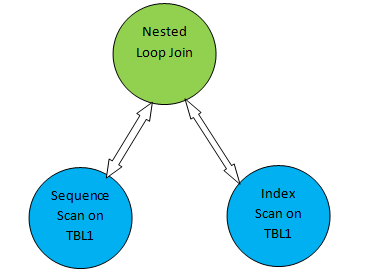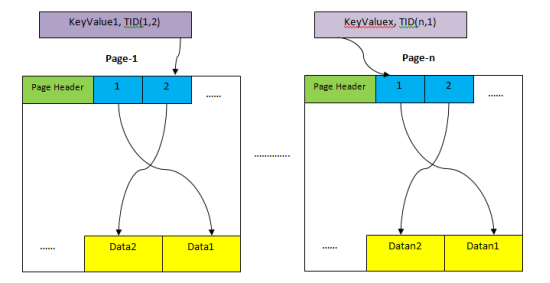PostgreSQL扫描方法综述

PostgreSQL 扫描方法综述HEAP ：存储表整个行的存储域。如上所示，整个域被分割为多个页，每个页大小默认是8K 。每个页中， item 指针（例如上述页中的 1,2 ）指向页内的数据。

Index Storage ：只存储KEY 值，即索引中包含的列值。也是分割成多个页，每个索引页默认 8K

Tuple Identifier(TID ) TID 6 个字节，包含两部分。前 4 个字节为页号，后 2 个字节为页内 tuple 索引。 TID 可以定位到特定记录。

postgres=# CREATE TABLE demotable (num numeric, id int);
CREATE TABLE
postgres=# CREATE INDEX demoidx ON demotable(num);
CREATE INDEX
postgres=# INSERT INTO demotable SELECT random() * 1000,  generate_series(1, 1000000);
INSERT 0 1000000
postgres=# analyze;
ANALYZE

postgres=# explain SELECT * FROM demotable WHERE num < 21000;
QUERY PLAN
--------------------------------------------------------------------
Seq Scan on demotable  (cost=0.00..17989.00 rows=1000000 width=15)
Filter: (num < '21000'::numeric)
(2 rows)

postgres=# explain SELECT * FROM demotable WHERE num = 21000;
QUERY PLAN
--------------------------------------------------------------------------
Index Scan using demoidx on demotable  (cost=0.42..8.44 rows=1 width=15)
Index Cond: (num = '21000'::numeric)
(2 rows)

Index Only Scan

postgres=# explain SELECT num FROM demotable WHERE num = 21000;
QUERY PLAN
-----------------------------------------------------------------------------
Index Only Scan using demoidx on demotable  (cost=0.42..8.44 rows=1 Width=11)
Index Cond: (num = '21000'::numeric)
(2 rows)

Bitmap Scan

Bitmap index scan ：首先获取索引数据并为所有 TID 创建 bitmap 。为了理解方法，可以认为 bitmap 包含所有页的哈希（基于页号），每个页的 entry 包含页内所有偏移的数组。

Bitmap heap scan ：从页的 bitmap 中读取值，然后针对页和偏移扫描数据。最后检查可见性和条件并返回 tuple

postgres=# explain SELECT * FROM demotable WHERE num < 210;
QUERY PLAN
--------------------------------------------------------------------------
Bitmap Heap Scan on demotable  (cost=5883.50..14035.53 rows=213042 width=15)
Recheck Cond: (num < '210'::numeric)
->  Bitmap Index Scan on demoidx  (cost=0.00..5830.24 rows=213042 width=0)
Index Cond: (num < '210'::numeric)
(4 rows)

postgres=# explain SELECT num FROM demotable WHERE num < 210;
QUERY PLAN
---------------------------------------------------------------------------
Index Only Scan using demoidx on demotable  (cost=0.42..7784.87 rows=208254 width=11)
Index Cond: (num < '210'::numeric)
(2 rows)

TID Scan

TID 扫描是 PG 中非常特殊的一种方式 , Oracle 中的基于 ROWID 查询类似：

postgres=# select ctid from demotable where id=21000;
ctid
----------
(115,42)
(1 row)
postgres=# explain select * from demotable where ctid='(115,42)';
QUERY PLAN
----------------------------------------------------------
Tid Scan on demotable  (cost=0.00..4.01 rows=1 width=15)
TID Cond: (ctid = '(115,42)'::tid)
(2 rows)

https://severalnines.com/database-blog/overview-various-scan-methods-postgresql

• 博文量
81
• 访问量
56391